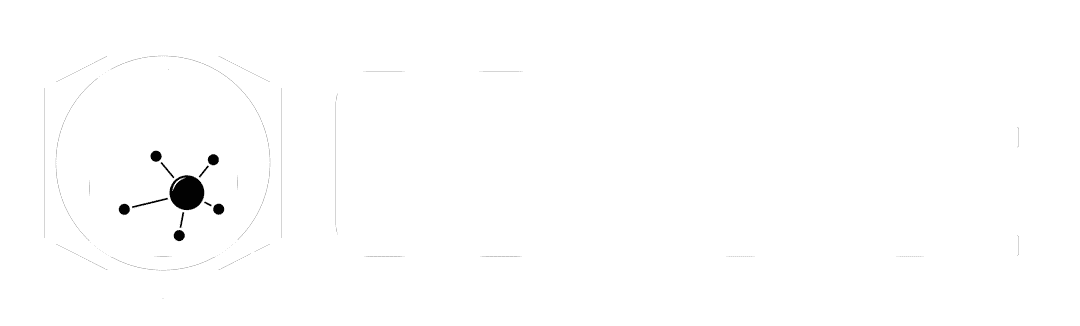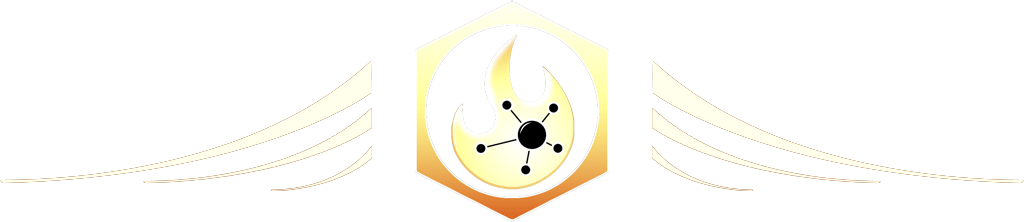# Resample

The Ultimate Houdini node reference

# Visit the Node Bible

### Resample

#### The Resample SOP resamples one or more curves or surfaces into even length segments.

Drag to resize

Summary:

The resample sop is very useful when you want to re-topologize a curve and/or the edge of a mesh into even segments.  This can apply to all curve types - including polygons, NURBS, or Bezier curves.  If you insert NURBS or Bezier curves, then the resample SOP will use a polygonal approximation to calculate the length of the curve and apply the results back onto the NURBS and Bezier curve.  This is important to understand because some parameters deal with this polygon to NURBS/Bezier process.

Drag to resize

Main Parameters:

Group:

- Allows you to isolate the resample to a certain group of points/primitives.  Read up on the Group SOP node bible entry if you're not familiar with groups yet.

Level of Detail:

--  If you feed the resample SOP a Bezier or NURBS curve, this "level of detail" refers to the level of detail that ought to be calculated for the incoming Bezier or NURBS curve.  If you're finding that the shape isn't quite right with an incoming NURBS or Bezier curve, then turn this up.  This will be especially noticeable in areas where the direction changes abruptly.

Resample by Polygon Edge:

--  This setting will help you preserve corners and sharp turns by sampling between poly edges.  In other words, the original point positions are preserved and any subdivision will happen between existing points.  Keep in mind that you may not get even spacing between points if you go this route.  In return though, your corners behave much better.

Method:

--  Even Length Segments = each segment has even spacing.
--  Even X,Y, and Z spacing = When determining the point spacing, look at the x,y, or z direction and make the splits even going in that particular direction.

Measure:

--  Along Arc = Tells the Resample SOP to use the points in space that originally defined the curve as the basis for rebuilding the curve.  When you use this mode, it will unlock the ability to use "Even Last Segment Same Length."  Another practical difference between Along Arc and Along Chord is if you turn off the Maximum Segment Length parameter.  When this is off, you can use the Maximum segments to define how many divisions you'd like in the curve.  If the measure is set to "Along Chord" however, you will not be able to use the "segments" to control divisions.
--  Along Chord = When using "Along Chord" you will not have access to "Even Last Segment Same Length."  This creates segments by measuring the distance between re-sampled points.  In practice, you will not notice much of a difference between Along Chord and Along Arc.  The main difference in functionality is the ability to control quality using the maximum segments if "Maximum Segment Length" is turned off.

Maximum Segment Length:

--  This parameter defines the distance between each segment.  Lower vals = more detail.  Higher values = less detail.

Maximum Segments:

--  This caps the number of segments which are allowed to define the curve.

Allow Primitive Attributes to Override Parameters:

--  If you feed the resample sop geometry which contains @segment_length or @num_segments, then the Maximum segment length and maximum segments will be controlled by those attributes.

Even Last Segment Same Length

--  This will make sure that the last segment is the same length as everything else.

Maintain Last Vertex:

--  This will force the resample to keep the last point of the curve.  If the curve doesn't have enough segments to reach the end of the curve, then it will just draw a straight line to the last point.  If you turn off "Maintain Last Vertex," then that will give you the ability to trim the ends of the curve.

Randomize First Segment Length:

--  This randomizes the length of the first segment.  According to the docs, "This option can help to eliminate aliasing when using the resample SOP to convert curves to a point cloud."

Create Only Points:

-- Does just like it says

Treat Polygon As:

--  If you have polylines or polygons, and you'd like to smoothen the flow of those polylines or polygon edges, then you can set this to Subdivision Curves or Interpolating Curves.  Subdivision Curves may cause volume loss.  Interpolating Curves may cause the edges to expand outwards.

Output as Polygon Curves to be Subdivided Later:

--  This will attempt to push points outward in a way that predicts the impact of subdivision smoothing at render.  If you want to subdivide the curve at render while maintaining your sharper corners, then try turning this on.

Distance Attribute:

--  According to the docs, "Creates a point attribute storing the length of output curve that is closest to each point along the curve. This is half the sum of the lengths of all edges connected to each output point. When creating only points, this value can be used to determine how much of the original curve each point represents."  In practice, it's unclear what this may get used for.

Tangent Attribute:

--  This attribute is very useful because it creates a vector attribute which points in the direction that a point is traveling along the curve.  So, if you want a vector attribute that's pointing along the direction of a curve, you can get this attribute with @tangentu

Curve U Attribute:

-- When the new curve is being generated, think of the beginning of that curve as a value of 0 and the end of the curve as a value of 1.  The CurveU attribute will figure out how far along the curve a particular point is.  If the value is .72, then it's basically like telling you, "This point is 72% along the original curve."  This is useful for control effects which rely on how far along the curve a point is.  Put another way - 0% says, "Hey, I'm at the beginning of the curve" and 100% says, "Hey, I'm at the end of the curve now."

Curve Number Attribute:

--  This creates a point attribute storing the curve primitive number for the resampled point.  In practice, it's like giving your curves a unique id.  If the resample generates four different curves, then the first one will have a curvenum of 0, the second curve will be 1, and so on.